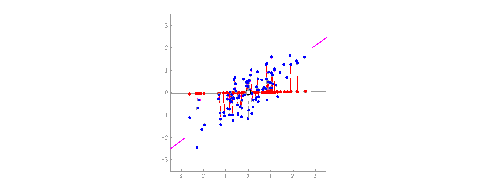Data can tell us stories. That’s what I’ve been told anyway. As a Data Scientist working for Fortune 300 clients, I deal with tons of data daily, I can tell you that data can tell us stories. You can apply a regression, classification or a clustering algorithm on the data, but feature selection and engineering can be a daunting task. A lot of times, I have seen data scientists take an automated approach to feature selection such as Recursive Feature Elimination (RFE) or leverage Feature Importance algorithms using Random Forest or XGBoost. All of these can be great methods, but may not be the best methods to get the “essence” of all of the data.

### Understanding the Nuances of PCA

#### The intuition of PCA

If we have two columns representing the X and Y columns, you can represent it in a 2D axis. Let’s say we add another dimension i.e., the Z-Axis, now we have something called a hyperplane representing the space in this 3D space.
Now, a dataset containing n-dimensions cannot be visualized as well.Re-align the axis to capture the most variance in the data

The idea of PCA is to re-align the axis in an n-dimensional space such that we can capture most of the variance in the data. In the industry, features that do not have much variance are discarded as they do not contribute much to any machine learning model. These new axes that represent most of the variance in the data are known as principal components.

The reason principal components are used is to deal with correlated predictors (multicollinearity) and to visualize data in a two-dimensional space.

PCA is a statistical procedure to convert observations of possibly correlated features to principal components such that:

• They are uncorrelated with each other
• They are linear combinations of original variables
• They help in capturing maximum information in the data set

PCA is the change of basis in the data.

#### Variance in PCA

If a column has less variance, it has less information. PCA changes the basis in such a way that the new basis vectors capture the maximum variance or information. These new basis vectors are known as Principal Components.

#### PCA as a dimensionality reduction technique

Imagine this situation that a lot of data scientists face. You have received the data, performed data cleaning, missing value analysis, data imputation. You now proceed to analyze the data further, notice the categorical columns and perform one-hot encoding on the data by making dummy variables. Now, we proceed to feature engineering and make even more features. I have had experiences where this leads to over 500, sometimes 1000 features.

Running Jupyter Notebook on the Cloud in 15 mins #Azure

How am I supposed to input so many features into a model or how am I supposed to know the important features? If we proceed to use Recursive Feature elimination or Feature Importance, I will be able to choose the columns that contribute the maximum to the expected output. However, what if we miss out on a feature that could contribute more to the model. The process of model iterations is error-prone and cumbersome. PCA is an alternative method we can leverage here.

Principal Component Analysis is a classic dimensionality reduction technique used to capture the essence of the data. It can be used to capture over 90% of the variance of the data.

Note: Variance does not capture the inter-column relationships or the correlation between variables. We perform diagonalization on the covariance matrix to obtain basis vectors that are:

• Uncorrelated
• Linearly Dependent
• Explain directions of maximum variance

The algorithm of PCA seeks to find new basis vectors that diagonalize the covariance matrix. This is done using Eigen Decomposition.

#### The Algorithm of PCA

1. Represent all the information in the dataset as a covariance matrix.
2. Perform Eigen Decomposition on the covariance matrix.
3. The new basis is the Eigenvectors of the covariance matrix obtained in Step I.
4. Represent the data on the new basis. The new basis is also called the principal components.

#### Code

Now, the articles I write here cannot be written without getting hands-on experience with coding. I believe your code should be where it belongs, not on Medium, but rather on GitHub.

anishmahapatra01/masters-machine-learning-1

I have laid out the commented code along with a sample clustering problem using PCA, along with the steps necessary to help you get started.

The logical steps are detailed out as shown below:

• Once the missing value and outlier analysis is complete, standardize/ normalize the data to help the model converge better
• We use the PCA package from sklearn to perform PCA on numerical and dummy features
• Use pca.components_ to view the PCA components generated
• Use PCA.explained_variance_ratio_ to understand what percentage of variance is explained by the data
• Scree plot is used to understand the number of principal components needs to be used to capture the desired variance in the data
• Run the machine-learning model to obtain the desired result